# Skewness and Kurtosis in R Programming

In statistics, skewness and kurtosis are the measures which tell about the shape of the data distribution or simply, both are numerical methods to analyze the shape of data set unlike, plotting graphs and histograms which are graphical methods. These are normality tests to check the irregularity and asymmetry of the distribution. To calculate skewness and kurtosis in R language, moments package is required.

#### Skewness

Skewness is a statistical numerical method to measure the asymmetry of the distribution or data set. It tells about the position of the majority of data values in the distribution around the mean value.

Formula:where,represents coefficient of skewnessrepresentsvalue in data vectorrepresents mean of data vector
n represents total number of observations

There exist 3 types of skewness values on the basis of which asymmetry of the graph is decided. These are as follows:

#### Positive Skew

If the coefficient of skewness is greater than 0 i.e., then the graph is said to be positively skewed with the majority of data values less than mean. Most of the values are concentrated on the left side of the graph.

Example:

 # Required for skewness() function  library(moments)     # Defining data vector  x <- c(40, 41, 42, 43, 50)     # output to be present as PNG file  png(file = "positiveskew.png")     # Print skewness of distribution  print(skewness(x))     # Histogram of distribution  hist(x)     # Saving the file  dev.off()

Output:

 1.2099


Graphical Representation: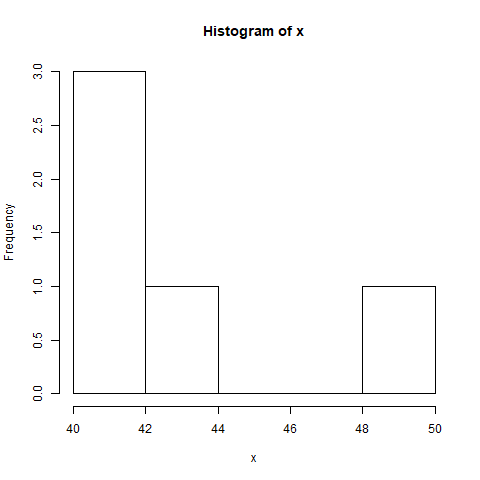#### Zero Skewness or Symmetric

If the coefficient of skewness is equal to 0 or approximately close to 0 i.e., then the graph is said to be symmetric and data is normally distributed.

Example:

 # Required for skewness() function  library(moments)     # Defining normally distributed data vector  x <- rnorm(50, 10, 10)     # output to be present as PNG file  png(file = "zeroskewness.png")     # Print skewness of distribution  print(skewness(x))     # Histogram of distribution  hist(x)     # Saving the file  dev.off()

Output:

 -0.02991511


Graphical Representation: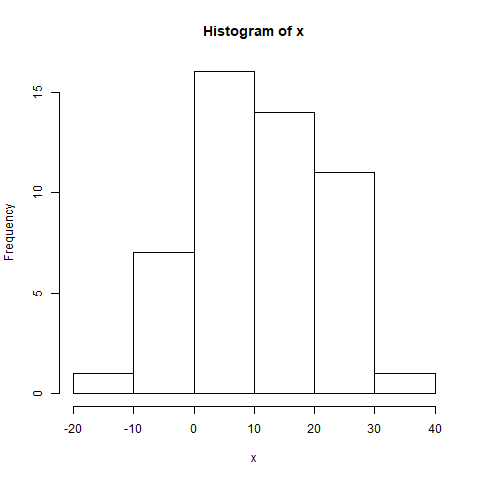#### Negatively skewed

If the coefficient of skewness is less than 0 i.e., then the graph is said to be negatively skewed with the majority of data values greater than mean. Most of the values are concentrated on the right side of the graph.

Example:

 # Required for skewness() function  library(moments)     # Defining data vector  x <- c(10, 11, 21, 22, 23, 25)     # output to be present as PNG file  png(file = "negativeskew.png")     # Print skewness of distribution  print(skewness(x))     # Histogram of distribution  hist(x)     # Saving the file  dev.off()

Output:

 -0.5794294


Graphical Representation: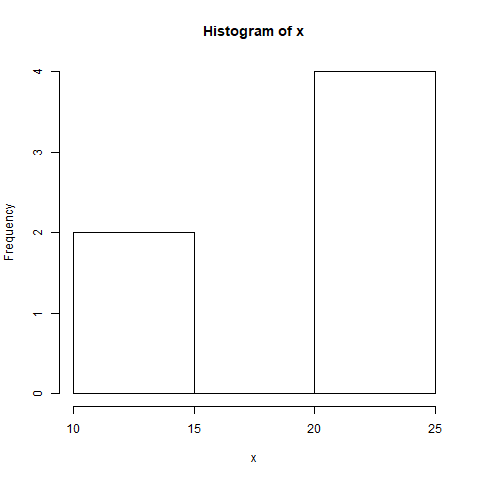#### Kurtosis

Kurtosis is a numerical method in statistics that measures the sharpness of the peak in the data distribution.

Formula:where,represents coefficient of kurtosisrepresentsvalue in data vectorrepresents mean of data vector
n represents total number of observations

There exist 3 types of Kurtosis values on the basis of which sharpness of the peak is measured. These are as follows:

#### Platykurtic

If the coefficient of kurtosis is less than 3 i.e., then the data distribution is platykurtic. Being platykurtic doesn’t mean that the graph is flat-topped.

Example:

 # Required for kurtosis() function  library(moments)     # Defining data vector  x <- c(rep(61, each = 10), rep(64, each = 18),   rep(65, each = 23), rep(67, each = 32), rep(70, each = 27),  rep(73, each = 17))     # output to be present as PNG file  png(file = "platykurtic.png")     # Print skewness of distribution  print(kurtosis(x))     # Histogram of distribution  hist(x)     # Saving the file  dev.off()

Output:

 2.258318


Graphical Representation: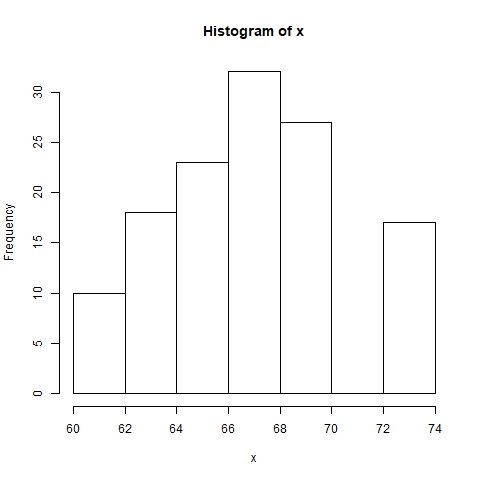#### Mesorkurtic

If the coefficient of kurtosis is equal to 3 or approximately close to 3 i.e., then the data distribution is mesokurtic. For normal distribution, kurtosis value is approximately equal to 3.

Example:

 # Required for kurtosis() function  library(moments)     # Defining data vector  x <- rnorm(100)     # output to be present as PNG file  png(file = "mesokurtic.png")     # Print skewness of distribution  print(kurtosis(x))     # Histogram of distribution  hist(x)     # Saving the file  dev.off()

Output:

 2.963836


Graphical Representation: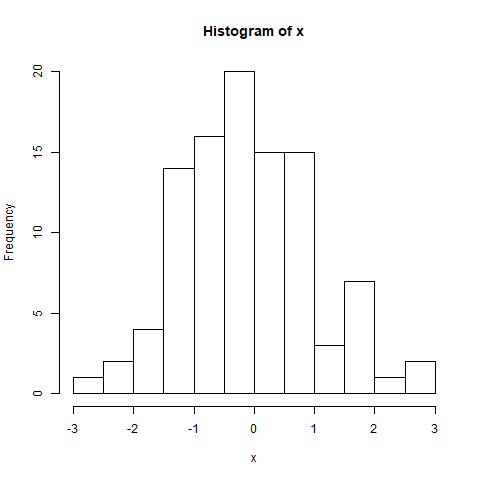#### Leptokurtic

If the coefficient of kurtosis is greater than 3 i.e., then the data distribution is leptokurtic and shows a sharp peak on the graph.

Example:

 # Required for kurtosis() function  library(moments)     # Defining data vector  x <- c(rep(61, each = 2), rep(64, each = 5),  rep(65, each = 42), rep(67, each = 12), rep(70, each = 10))     # output to be present as PNG file  png(file = "leptokurtic.png")     # Print skewness of distribution  print(kurtosis(x))     # Histogram of distribution  hist(x)     # Saving the file  dev.off()

Output:

 3.696788


Graphical Representation: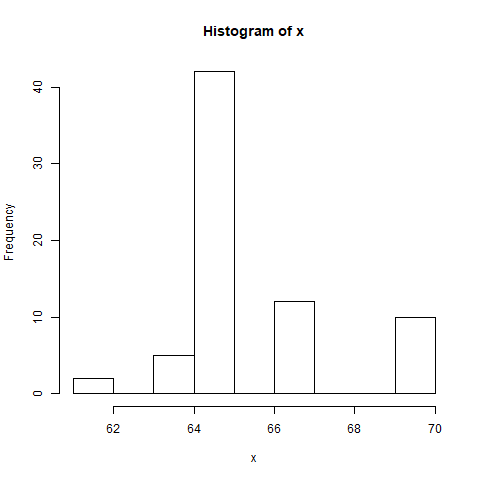My Personal Notes arrow_drop_upCheck out this Author's contributed articles.

If you like GeeksforGeeks and would like to contribute, you can also write an article using contribute.geeksforgeeks.org or mail your article to contribute@geeksforgeeks.org. See your article appearing on the GeeksforGeeks main page and help other Geeks.

Please Improve this article if you find anything incorrect by clicking on the "Improve Article" button below.

Article Tags :

1

Please write to us at contribute@geeksforgeeks.org to report any issue with the above content.